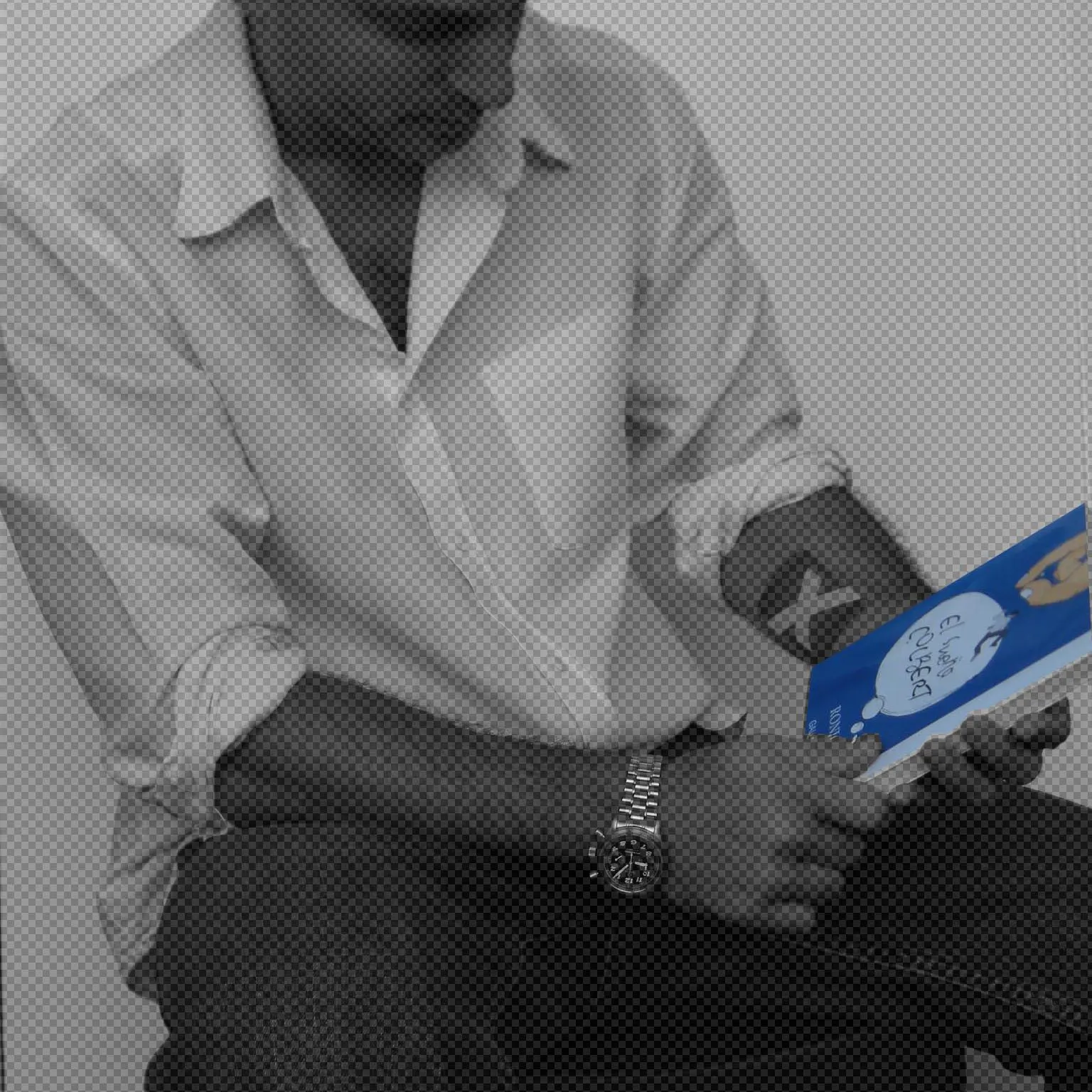# Book Signer

Are you a writer facing a book signing? Don't worry, this machine will do it for you.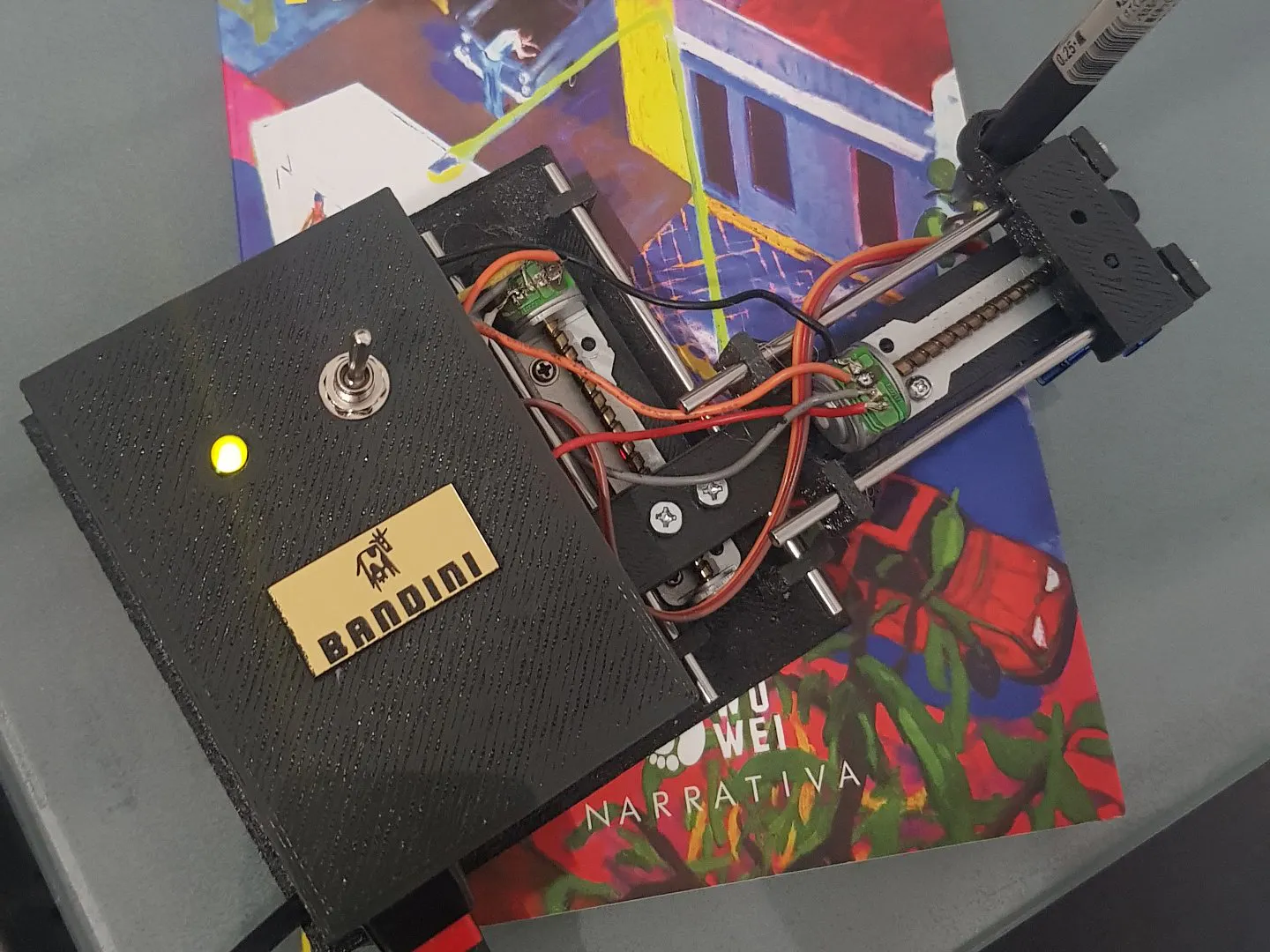## Things used in this project

### Hardware componentsArduino UNO
×1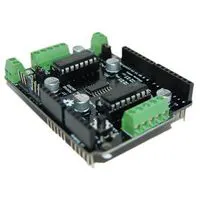Development Board, Motor Control Shield
×1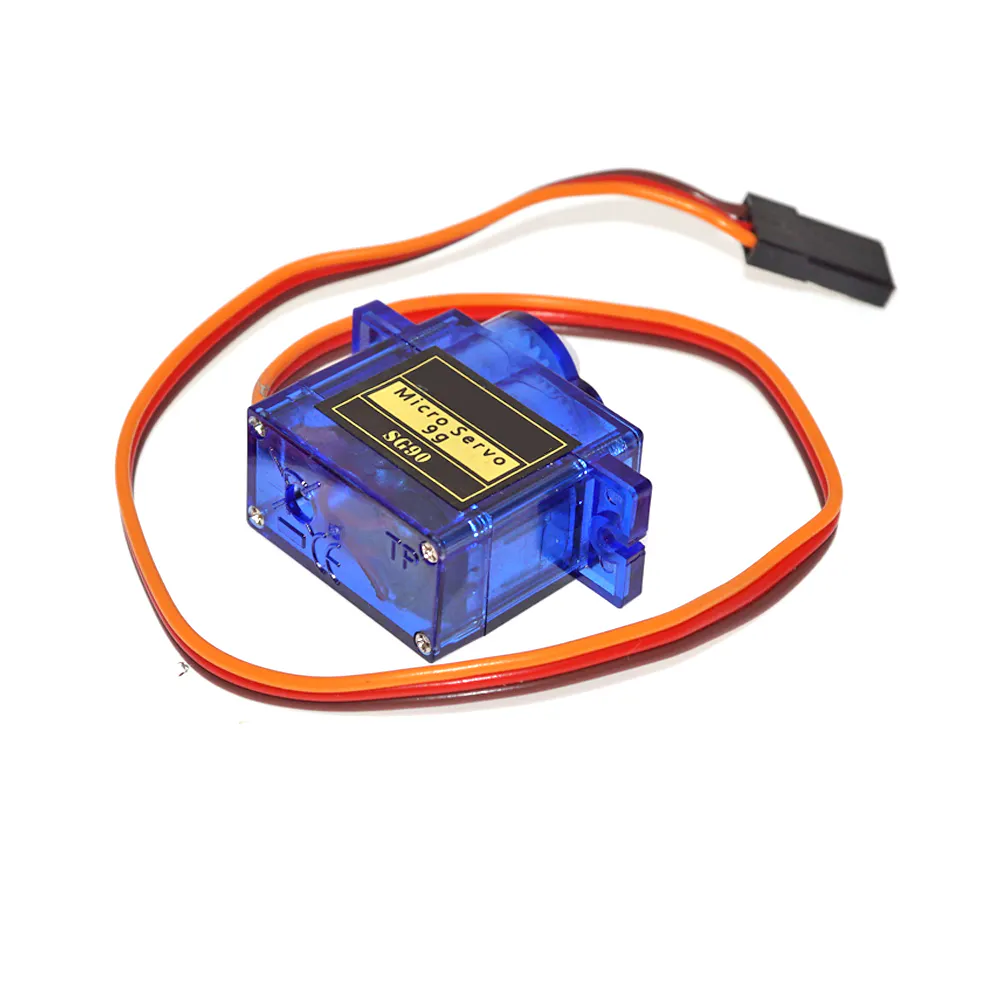SG90 Micro-servo motor
×1LED (generic)
×1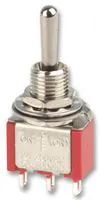Toggle Switch, (On)-Off-(On)
×1

### Software apps and online servicesArduino IDE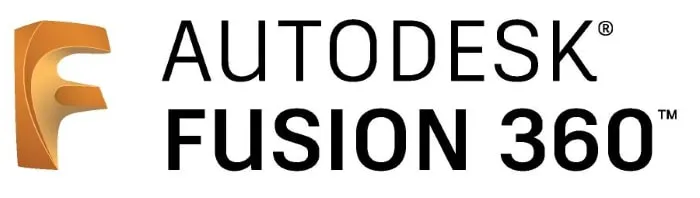Autodesk Fusion 360

## Code

### Book Signer

C/C++
```/*
Book Signer
Roni Bandini
Based in TinyCNC https://github.com/MakerBlock/TinyCNC-Sketches

*/
#include <Servo.h>
#include <AFMotor.h>
#define LINE_BUFFER_LENGTH 512

char STEP = MICROSTEP ;

// To lift pen with SG90
const int penZUp = 90;
const int penZDown = 110;
const int penServoPin =9 ;
const int ledPin=2;
const int stepsPerRevolution = 200;

Servo penServo;

// Motors connected to Arduino Shield motors 1 and 2, leave middle screw without cable
AF_Stepper myStepperY(stepsPerRevolution,1);
AF_Stepper myStepperX(stepsPerRevolution,2);

struct point {
float x;
float y;
float z;
};

struct point actuatorPos;

//  Drawing settings
float StepInc = 1;
int StepDelay = 1;
int LineDelay = 1;
int penDelay = 50;

// Motor steps to go 1 millimeter.
// Use test sketch to go 100 steps. Measure the length of line.
// Calculate steps per mm. Enter here.
float StepsPerMillimeterX = 100.0;
float StepsPerMillimeterY = 100.0;

// Drawing robot limits, in mm
float Xmin = 0;
float Xmax = 150;
float Ymin = 0;
float Ymax = 150;
float Zmin = 0;
float Zmax = 1;
float Xpos = Xmin;
float Ypos = Ymin;
float Zpos = Zmax;

// Set to true to get debug output.
boolean verbose = false;

void setup() {
//  Setup

Serial.begin(9600);

pinMode(ledPin, OUTPUT);

digitalWrite(ledPin, HIGH);
delay(200);
digitalWrite(ledPin, LOW);
delay(200);
digitalWrite(ledPin, HIGH);

penServo.attach(penServoPin);
penServo.write(penZUp);
delay(100);

myStepperX.setSpeed(100);
myStepperY.setSpeed(100);
myStepperX.release();
myStepperY.release();

Serial.println("Book Signer - Roni Bandini");

}
/**********************
* void loop() - Main loop
***********************/
void loop()
{

delay(100);

char line[ LINE_BUFFER_LENGTH ];
char c;
int lineIndex;
bool lineIsComment, lineSemiColon;
lineIndex = 0;
lineSemiColon = false;
lineIsComment = false;
while (1) {
// Serial reception - Mostly from Grbl, added semicolon support
while ( Serial.available()>0 ) {
if (( c == '\n') || (c == '\r') ) {             // End of line reached
if ( lineIndex > 0 ) {                        // Line is complete. Then execute!
line[ lineIndex ] = '\0';                   // Terminate string
if (verbose) {
Serial.println( line );
}
processIncomingLine( line, lineIndex );
lineIndex = 0;
}
else {
// Empty or comment line. Skip block.
}
lineIsComment = false;
lineSemiColon = false;
Serial.println("ok");
}
else {
if ( (lineIsComment) || (lineSemiColon) ) {   // Throw away all comment characters
if ( c == ')' )  lineIsComment = false;     // End of comment. Resume line.
}
else {
if ( c <= ' ' ) {                           // Throw away whitepace and control characters
}
else if ( c == '/' ) {                    // Block delete not supported. Ignore character.
}
else if ( c == '(' ) {                    // Enable comments flag and ignore all characters until ')' or EOL.
lineIsComment = true;
}
else if ( c == ';' ) {
lineSemiColon = true;
}
else if ( lineIndex >= LINE_BUFFER_LENGTH-1 ) {
Serial.println( "ERROR - lineBuffer overflow" );
lineIsComment = false;
lineSemiColon = false;
}
else if ( c >= 'a' && c <= 'z' ) {        // Upcase lowercase
line[ lineIndex++ ] = c-'a'+'A';
}
else {
line[ lineIndex++ ] = c;
}
}
}
}
}
}
void processIncomingLine( char* line, int charNB ) {
int currentIndex = 0;
char buffer[ 64 ];                                 // Hope that 64 is enough for 1 parameter
struct point newPos;
newPos.x = 0.0;
newPos.y = 0.0;

while( currentIndex < charNB ) {
switch ( line[ currentIndex++ ] ) {              // Select command, if any
case 'U':
digitalWrite(ledPin, LOW);
penUp();
break;
case 'D':
digitalWrite(ledPin, HIGH);
penDown();
break;
case 'G':
buffer = line[ currentIndex++ ];          // /!\ Dirty - Only works with 2 digit commands
//      buffer = line[ currentIndex++ ];
//      buffer = '\0';
buffer = '\0';
switch ( atoi( buffer ) ){                   // Select G command
case 0:                                   // G00 & G01 - Movement or fast movement. Same here
case 1:
// /!\ Dirty - Suppose that X is before Y
char* indexX = strchr( line+currentIndex, 'X' );  // Get X/Y position in the string (if any)
char* indexY = strchr( line+currentIndex, 'Y' );
if ( indexY <= 0 ) {
newPos.x = atof( indexX + 1);
newPos.y = actuatorPos.y;
}
else if ( indexX <= 0 ) {
newPos.y = atof( indexY + 1);
newPos.x = actuatorPos.x;
}
else {
newPos.y = atof( indexY + 1);
indexY = '\0';
newPos.x = atof( indexX + 1);
}
drawLine(newPos.x, newPos.y );
//        Serial.println("ok");
actuatorPos.x = newPos.x;
actuatorPos.y = newPos.y;
break;
}
break;
case 'M':
buffer = line[ currentIndex++ ];        // /!\ Dirty - Only works with 3 digit commands
buffer = line[ currentIndex++ ];
buffer = line[ currentIndex++ ];
buffer = '\0';
switch ( atoi( buffer ) ){
case 300:
{
char* indexS = strchr( line+currentIndex, 'S' );
float Spos = atof( indexS + 1);
//         Serial.println("ok");
if (Spos == 30) {
penDown();
}
if (Spos == 50) {
penUp();
}
break;
}
case 114:                                // M114 - Repport position
Serial.print( "Absolute position : X = " );
Serial.print( actuatorPos.x );
Serial.print( "  -  Y = " );
Serial.println( actuatorPos.y );
break;
default:
Serial.print( "Command not recognized : M");
Serial.println( buffer );
}
}
}
}

void drawLine(float x1, float y1) {
if (verbose)
{
Serial.print("fx1, fy1: ");
Serial.print(x1);
Serial.print(",");
Serial.print(y1);
Serial.println("");
}
//  Bring instructions within limits
if (x1 >= Xmax) {
x1 = Xmax;
}
if (x1 <= Xmin) {
x1 = Xmin;
}
if (y1 >= Ymax) {
y1 = Ymax;
}
if (y1 <= Ymin) {
y1 = Ymin;
}
if (verbose)
{
Serial.print("Xpos, Ypos: ");
Serial.print(Xpos);
Serial.print(",");
Serial.print(Ypos);
Serial.println("");
}
if (verbose)
{
Serial.print("x1, y1: ");
Serial.print(x1);
Serial.print(",");
Serial.print(y1);
Serial.println("");
}
//  Convert coordinates to steps
x1 = (int)(x1*StepsPerMillimeterX);
y1 = (int)(y1*StepsPerMillimeterY);
float x0 = Xpos;
float y0 = Ypos;
//  Let's find out the change for the coordinates
long dx = abs(x1-x0);
long dy = abs(y1-y0);
int sx = x0<x1 ? StepInc : -StepInc;
int sy = y0<y1 ? StepInc : -StepInc;
long i;
long over = 0;
if (dx > dy) {
for (i=0; i<dx; ++i) {
myStepperX.onestep(sx,STEP);
over+=dy;
if (over>=dx) {
over-=dx;
myStepperY.onestep(sy,STEP);
}
delay(StepDelay);
}
}
else {
for (i=0; i<dy; ++i) {
myStepperY.onestep(sy,STEP);
over+=dx;
if (over>=dy) {
over-=dy;
myStepperX.onestep(sx,STEP);
}
delay(StepDelay);
}
}
if (verbose)
{
Serial.print("dx, dy:");
Serial.print(dx);
Serial.print(",");
Serial.print(dy);
Serial.println("");
}
if (verbose)
{
Serial.print("Going to (");
Serial.print(x0);
Serial.print(",");
Serial.print(y0);
Serial.println(")");
}
//  Delay before any next lines are submitted
delay(LineDelay);
//  Update the positions
Xpos = x1;
Ypos = y1;
}
//  Raises pen
void penUp() {
penServo.write(penZUp);
delay(penDelay);
Zpos=Zmax;
digitalWrite(15, LOW);
digitalWrite(16, HIGH);
if (verbose) {
Serial.println("Pen up!");

}
}
//  Lowers pen
void penDown() {
penServo.write(penZDown);
delay(penDelay);
Zpos=Zmin;
digitalWrite(15, HIGH);
digitalWrite(16, LOW);
if (verbose) {
Serial.println("Pen down.");

}
}
```

## Credits

### Roni Bandini

36 projects • 102 followers
Writer, electronic artist and musician from Argentina